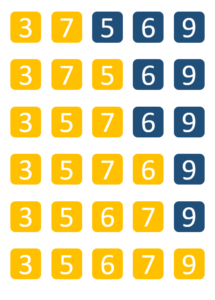# How to Implement Insertion Sort in Java?Insertion Sort in java is a simple and efficient sorting algorithm, that creates the final sorted array one element at a time. It is usually implemented when the user has a small data set. I’ll cover the following topics:

## What is Insertion Sort?

Insertion Sort in java is an efficient sorting algorithm, that creates the final sorted array one element at a time. An element from the input data is removed after every iteration. It is compared to the largest value present in the array and is then moved to the correct position. To understand the working of this sort lets take a look at this example.## Algorithm of Insertion Sort

Let’s say we have an unsorted array [6, 5, 15, 3, 9]

• 1st index iteration: The value at the 1st index is 5, which is less than 6. The array becomes [6, 6, 15, 2, 8].

On reaching the start of the set of elements,  we place the value at the 0th index.The array now becomes: [5, 6, 15, 3, 9]

• 2nd index iteration: The value at the 2nd index is 15,  which is greater than 6. No changes are made in the array.

• 3rd index iteration:  The value at the 3rd index is 3. The value is lesser than 15, thus the array becomes [5, 6, 15, 15, 9]

The value 3 is also lesser than 6, thus the array now changes to [5, 6, 6, 15, 9]

3 is smaller than 5 as well. The array is again modified to [5, 5, 6, 15, 9]

When the beginning of the array is reached, 3 is placed at the 0th index. The array is now defined as [3, 5, 6, 15, 9]

• 4th index iteration: The value at the 4th index is 9. Following a similar algorithm, the final sorted array is : [3, 5, 6, 9, 15]

## Code for Insertion Sort in Java

```// Java program to implement Insertion Sort
public class InsertionEx {
/*Function to sort array using insertion sort*/
void sort(int a[])
{
int n = a.length;
for (int i = 1; i < n; ++i) { int key = a[i]; int j = i - 1; /* Move elements of a[0..i-1], that are greater than key, to one position ahead of their current position */ while (j >= 0 && a[j] > key) {
a[j + 1] = a[j];
j = j - 1;
}
a[j + 1] = key;
}
}

/* A function to print array of size n*/
static void displayArray(int a[])
{
int n = a.length;
for (int i = 0; i < n; ++i)
System.out.print(a[i] + " ");

System.out.println();
}

// Driver code
public static void main(String args[])
{
int a[] = { 25, 28, 18, 10, 5 };

InsertionEx ob = new InsertionEx();
ob.sort(a);
displayArray(a);
}
}```

## Complexity and Boundary Cases

• Time Complexity: The time complexity of insertion sort is O(n*2).

• Boundary Cases: The maximum time taken by the insertion sort is when the elements are sorted in the reverse order. If the elements are already sorted, it takes minimum time

Insertion Sort is implemented by the user when the number of elements to be sorted is less in number. It can also be used when the array specified is almost sorted i.e. only a few numbers are misplaced and not in the appropriate positions.

With this, we come to an end of this Insertion Sort in Java article. Check out the Java training by Edureka, a trusted online learning company with a network of more than 250,000 satisfied learners spread across the globe. Edureka’s Java J2EE and SOA training and certification course is designed for students and professionals who want to be a Java Developer. The course is designed to give you a head start into Java programming and train you for both core and advanced Java concepts along with various Java frameworks like Hibernate & Spring.

Got a question for us? Please mention it in the comments section of this “Insertion Sort in Java” blog and we will get back to you as soon as possible.

Upcoming Batches For Java Certification Training Course
Course NameDate
Java Certification Training Course

Class Starts on 7th October,2023

7th October

SAT&SUN (Weekend Batch)
View Details
Java Certification Training Course

Class Starts on 25th November,2023

25th November

SAT&SUN (Weekend Batch)
View DetailsREGISTER FOR FREE WEBINARThank you for registering Join Edureka Meetup community for 100+ Free Webinars each month